Next: Inference for simple hypotheses Up: Bayesian inference for simple Previous: Background information

## Bayes' theorem

Formally, Bayes' theorem follows from the symmetry of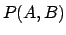expressed by Eq. (17). In terms of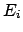and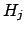belonging to two different complete classes, Eq. (17) yields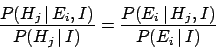(18)

This equation says that the new conditionalters our belief inby the same updating factor by which the conditionalters our belief about. Rearrangement yields Bayes' theorem(19)

We have obtained a logical rule to update our beliefs on the basis of new conditions. Note that, though Bayes' theorem is a direct consequence of the basic rules of axiomatic probability theory, its updating power can only be fully exploited if we can treat on the same basis expressions concerning hypotheses and observations, causes and effects, models and data.

In most practical cases, the evaluation of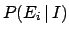can be quite difficult, while determining the conditional probability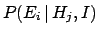might be easier. For example, think ofas the probability of observing a particular event topology in a particle physics experiment, compared with the probability of the same thing given a value of the hypothesized particle mass (), a given detector, background conditions, etc. Therefore, it is convenient to rewritein Eq. (19) in terms of the quantities in the numerator, using Eq. (13), to obtain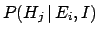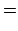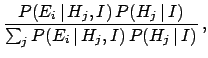(20)

which is the better-known form of Bayes' theorem. Written this way, it becomes evident that the denominator of the r.h.s. of Eq. (20) is just a normalization factor and we can focus on just the numerator: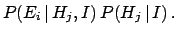(21)

In words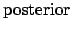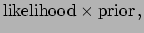(22)

where the posterior (or final state) stands for the probability of, based on the new observation, relative to the prior (or initial) probability. (Prior probabilities are often indicated with.) The conditional probabilityis called the likelihood. It is literally the probability of the observationgiven the specific hypothesis. The term likelihood can lead to some confusion, because it is often misunderstood to mean the likelihood thatcomes from.'' However, this name implies to considera mathematical function offor a fixedand in that framework it is usually written asto emphasize the functionality. We caution the reader that one sometimes even finds the notation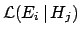to indicate exactly.Next: Inference for simple hypotheses Up: Bayesian inference for simple Previous: Background information
Giulio D'Agostini 2003-05-13